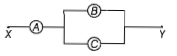# NEET Physics Current Electricity Questions Solved

A.B and C are voltmeters of resistance R,1.5R and 3R respectively as shown in the figure. When some potential difference is applied between X and Y. the voltmeter readings are VA, VB and VC respectively.Then,(a)VA=VB=VC

(b)VA≠VB=VC

(c)VA=VB≠VC

(d)VA≠VB≠VC